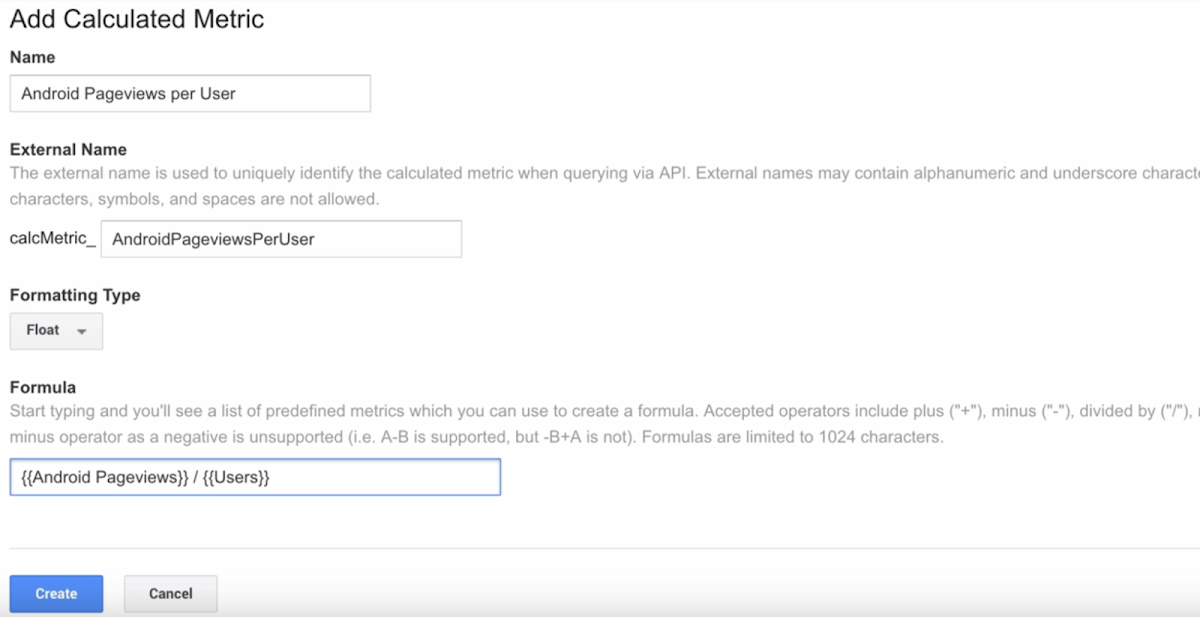# How to set up Calculated Metrics

### Overview

Calculated Metrics let you perform mathematical computations on existing metrics using basic addition, subtraction, multiplication, and division.

If the Google Merchandise Store wanted to see the Custom Metric “Android merchandise pageviews” divided by users, they can create a Calculated Metric that divides the number of pageviews by the number of users.

### Set up

To set up Calculated Metrics, go into Admin, and under Views click Calculated Metric. Then click New Calculated Metric.

We’ll name the Calculated Metric “Android Pageviews per User”. Note that Google Analytics automatically includes an external name for querying this calculated metric via the API. Since our metric will likely be a fraction, we’ll set the formatting type to “Float.”

If you start typing in the Formula field, you'll see a list of predefined metrics which you can use to create a formula. You can only use “plus,” “minus,” “divided by,” and “multiplied by.” You cannot, however, use the minus operator for negative numbers. Also be aware that formulas are limited to 1,024 characters at this time.To create our Calculated Metric we begin by entering the name of our custom metric: “Android pageviews.” Then, we enter the division sign. And finally the metric “users.” Then click Create.

Once you’ve created the Calculated Metric you can add it to Custom Reports, unsampled reports, or dashboard widgets.